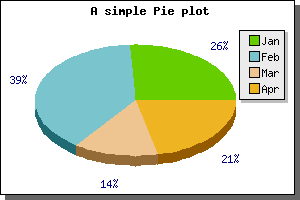Contents Previous Next

### 9.2.2 Creating 3D pie plots

So far we have only made use of 2D pie plots, creating 3D pie plots is no more difficult. Instead of creating the plots with a call to PiePlot() you create the plots with a call to PiePlot3D() If we just take the first simple pie plot and replace the call to PiePlot() with a call to PiePlot3D() we get the following result.Figure 123: A first example of a 3D pie plot [src]

3D Pie plots have the same possibilities as the normal pie plots with the added twist of a 3:rd dimension. You can adjust the perspective angle with the method SetAngle() So for example to make the pie more "flat" you just set it to a smaller angle. Setting the perspective angle to 20 degrees in the previous example will give the following result.Figure 124: Adjusting the perspective angle [src]

Contents Previous Next